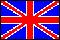ISSN 2079-3537

 × Scientific Visualization, 2018, volume 10, number 2, pages 95 - 111, DOI: 10.26583/sv.10.2.08Visualization of tensor fields based on geometric representation of tensorsAuthors: Yu.I. Dimitrienko1, E.A. Gubareva2, S.V. Sborshikov3Bauman Moscow State Technical University (BMSTU), Moscow, Russia 1 ORCID: 0000-0002-8610-8770, dimit@bmstu.ru 2 ORCID: 0000-0003-1678-3372, elena.a.gubareva@yandex.ru 3 ORCID: 0000-0001-5888-3019, servasbor@gmail.com  Abstract In comparison with scalar fields (temperature, density, pressure, etc.) and vector fields (velocity vector, displacement vector), the principles of 3D visualization of which are well developed at the present time, there are practically no methods of visualizing the fields of second-rank tensors. Therefore, for the basic tensor that characterizes the stress state in solids, the Cauchy stress tensor, at present, mainly component visualization is used, as a set of scalar fields, or symbolic 2D visualization, in which each tensor is considered an ellipse. In this paper, we present the results of developing a new method for visualizing tensor fields, both in two-dimensional and three-dimensional space, which uses the so-called geometric representation of second-rank tensors, in the form of an ordered set of 4 or 6 vectors, respectively. The idea and theoretical basis of the method was previously proposed by Yu.I. Dimitrienko. The results of the development of a specialized software module TensorView, which allows visualizing tensor fields using the geometric representation of tensors, are presented. Examples of the use of the visualization of tensor fields in the problem of modeling the stress-strain state of elements of aviation structures are presented. The proposed new method of visualization of tensor fields makes it possible to give an idea of tensors as integral invariant objects, generalizing well-known representations about vector fields in three-dimensional space.   Keywords: tensor, tensor field, geometric representation of tensors, visualization of tensor fields.